# 09-240/Classnotes for Tuesday September 15

WARNING: The notes below, written for students and by students, are provided "as is", with absolutely no warranty. They can not be assumed to be complete, correct, reliable or relevant. If you don't like them, don't read them. It is a bad idea to stop taking your own notes thinking that these notes can be a total replacement - there's nothing like one's own handwriting! Visit this pages' history tab to see who added what and when.

The real numbers A set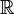$\mathbb R$ with two binary operators and two special elements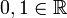$0, 1 \in \mathbb R$ s.t.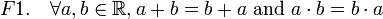$F1.\quad \forall a, b \in \mathbb R, a + b = b + a \mbox{ and } a \cdot b = b \cdot a$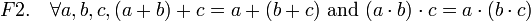$F2.\quad \forall a, b, c, (a + b) + c = a + (b + c) \mbox{ and } (a \cdot b) \cdot c = a \cdot (b \cdot c)$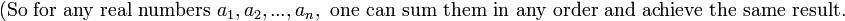$\mbox{(So for any real numbers } a_1, a_2, ..., a_n, \mbox{ one can sum them in any order and achieve the same result.}$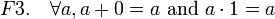$F3.\quad \forall a, a + 0 = a \mbox{ and } a \cdot 1 = a$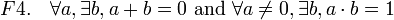$F4.\quad \forall a, \exists b, a + b = 0 \mbox{ and } \forall a \ne 0, \exists b, a \cdot b = 1$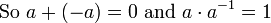$\mbox{So } a + (-a) = 0 \mbox{ and } a \cdot a^{-1} = 1$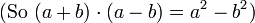$\mbox{(So } (a + b) \cdot (a - b) = a^2 - b^2)$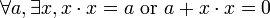$\forall a, \exists x, x \cdot x = a \mbox{ or } a + x \cdot x = 0$
Note: or means inclusive or in math.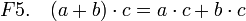$F5.\quad (a + b) \cdot c = a \cdot c + b \cdot c$

Definition: A field is a set F with two binary operators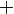$\,\!+$: F×FF,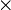$\times\,\!$: F×FF and two elements$0, 1 \in \mathbb R$ s.t.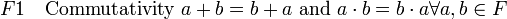$F1\quad \mbox{Commutativity } a + b = b + a \mbox{ and } a \cdot b = b \cdot a \forall a, b \in F$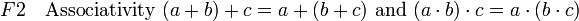$F2\quad \mbox{Associativity } (a + b) + c = a + (b + c) \mbox{ and } (a \cdot b) \cdot c = a \cdot (b \cdot c)$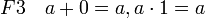$F3\quad a + 0 = a, a \cdot 1 = a$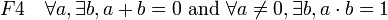$F4\quad \forall a, \exists b, a + b = 0 \mbox{ and } \forall a \ne 0, \exists b, a \cdot b = 1$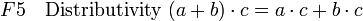$F5\quad \mbox{Distributivity } (a + b) \cdot c = a \cdot c + b \cdot c$

## Examples

1.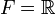$F = \mathbb R$
2.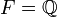$F = \mathbb Q$
3.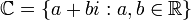$\mathbb C = \{ a + bi : a, b \in \mathbb R \}$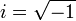$i = \sqrt{-1}$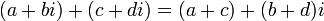$\,\!(a + bi) + (c + di) = (a + c) + (b + d)i$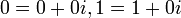$\,\!0 = 0 + 0i, 1 = 1 + 0i$
4.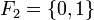$\,\!F_2 = \{ 0, 1 \}$
5.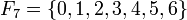$\,\!F_7 = \{ 0, 1,2,3,4,5,6 \}$
6.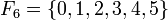$\,\!F_6 = \{ 0, 1,2,3,4,5 \}$ is not a field because not every element has a multiplicative inverse.
Let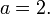$a = 2.$
Then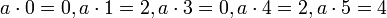$a \cdot 0 = 0, a \cdot 1 = 2, a \cdot 3 = 0, a \cdot 4 = 2, a \cdot 5 = 4$
Therefore F4 fails; there is no number b in F6 s.t. a · b = 1
Ex. 4
+ 0 1
0 0 1
1 1 0
Ex. 4
× 0 1
0 0 0
1 0 1
Ex. 5
+ 0 1 2 3 4 5 6
0 0 1 2 3 4 5 6
1 1 2 3 4 5 6 0
2 2 3 4 5 6 0 1
3 3 4 5 6 0 1 2
4 4 5 6 0 1 2 3
5 5 6 0 1 2 3 4
6 6 0 1 2 3 4 5
Ex. 5
× 0 1 2 3 4 5 6
0 0 0 0 0 0 0 0
1 0 1 2 3 4 5 6
2 0 2 4 6 1 3 5
3 0 3 6 2 5 1 4
4 0 4 1 5 2 6 3
5 0 5 3 1 6 4 2
6 0 6 5 4 3 2 1

Theorem: F2 is a field.

In order to prove that the associative property holds, make a table (similar to a truth table) for a, b and c.

a b c
0 0 0
0 0 1
0 1 0
0 1 1 (0 + 1) + 1 =? 0 + (1 + 1)
1 + 1 =? 0 + 0
0 = 0
1 0 0
1 0 1
1 1 0
1 1 1

Theorem: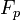$\,\! F_p$ for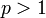$p > 1$ is a field iff (if and only if)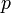$p$ is a prime number

Proof:

Given a finite set with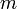$m$ elements in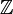$\mathbb Z$, an element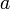$a$ will have a multiplicative inverse iff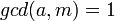$gcd(a,m) = 1$

This can be shown using Bézout's identity: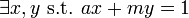$\exists x, y \mbox{ s.t. } ax + my = 1$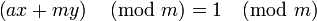$\left(ax + my\right) \pmod{m} = 1\pmod{m}$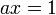$ax = 1$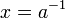$x = a^{-1}$

We have shown that$a$ has a multiplicative inverse if$a$ and$m$ are relatively prime. It is therefore a natural conclusion that if$m$ is prime all elements in the set will satisfy$gcd(a, m) = 1$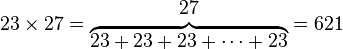$23 \times 27 = \begin{matrix} 27 \\ \overbrace{23 + 23 + 23 + \cdots + 23} \end{matrix} = 621$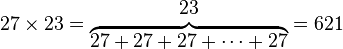$27 \times 23 = \begin{matrix} 23 \\ \overbrace{27 + 27 + 27 + \cdots + 27} \end{matrix} = 621$

One may interpret this as counting the units in a 23×27 rectangle; one may choose to count along either 23 rows or 27 columns, but both ways lead to the same answer.

You may also think of it as 27-n=23 23*23 + 23*n = 27*23. Exponentiation is repeated multiplication, but it does not have the same properties as multiplication; 23 = 8, but 32 = 9.

## Tedious Theorem

1.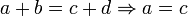$a + b = c + d \Rightarrow a = c$ "cancellation property"
Proof:
By F4,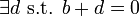$\exists d \mbox{ s.t. } b + d = 0$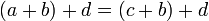$\,\! (a + b) + d = (c + b) + d$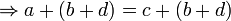$\Rightarrow a + (b + d) = c + (b + d)$ by F2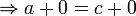$\Rightarrow a + 0 = c + 0$ by choice of d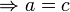$\Rightarrow a = c$ by F3
2.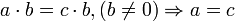$a \cdot b = c \cdot b , (b \ne 0) \Rightarrow a = c$
3.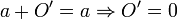$a + O' = a \Rightarrow O' = 0$
Proof: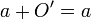$\,\! a + O' = a$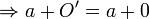$\Rightarrow a + O' = a + 0$ by F3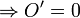$\Rightarrow O' = 0$ by adding the additive inverse of a to both sides
4.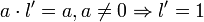$a \cdot l' = a, a \ne 0 \Rightarrow l' = 1$
5.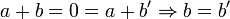$a + b = 0 = a + b' \Rightarrow b = b'$
6.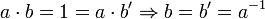$a \cdot b = 1 = a \cdot b' \Rightarrow b = b' = a^{-1}$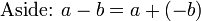$\,\! \mbox{Aside: } a - b = a + (-b)$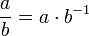$\frac ab = a \cdot b^{-1}$
7.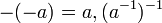$\,\! -(-a) = a, (a^{-1})^{-1}$
8.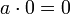$a \cdot 0 = 0$
Proof: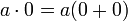$a \cdot 0 = a(0 + 0)$ by F3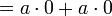$= a \cdot 0 + a \cdot 0$ by F5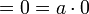$= 0 = a \cdot 0$
9.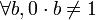$\forall b, 0 \cdot b \ne 1$
So there is no 0−1
10.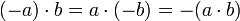$(-a) \cdot b = a \cdot (-b) = -(a \cdot b)$
11.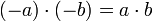$(-a) \cdot (-b) = a \cdot b$
12. (Bonus)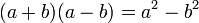$\,\! (a + b)(a - b) = a^2 - b^2$

......